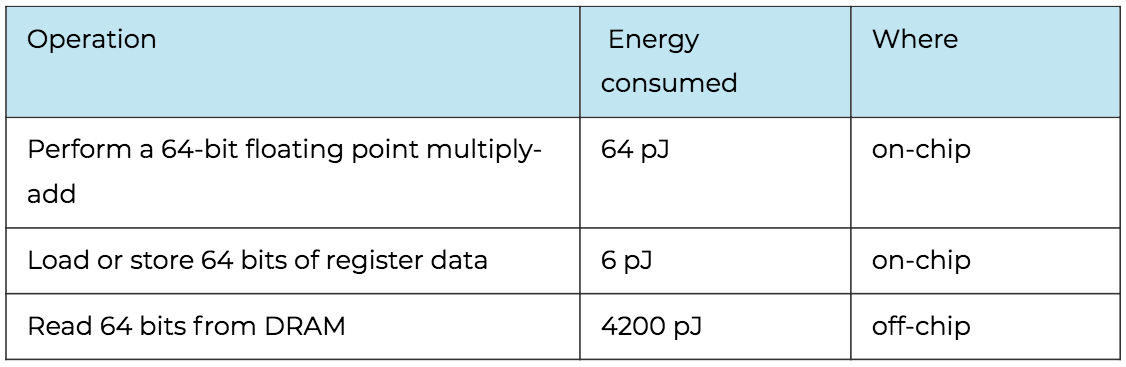Over a million developers have joined DZone.{{announcement.body}}
{{announcement.title}}

# The Quadratic Equation and Low-Precision Arithmetic

DZone 's Guide to

# The Quadratic Equation and Low-Precision Arithmetic

### There's an interesting wrinkle in the quadratic formula. Let's take a look at it and fix it.

· Big Data Zone ·
Free Resource

Comment (0)

Save
{{ articles.views | formatCount}} Views

What could be interesting about the lowly quadratic formula? It's a formula after all. You just stick numbers into it.

Well, there's an interesting wrinkle. When the linear coefficient b is large relative to the other coefficients, the quadratic formula can give wrong results when implemented in floating point arithmetic.

## Quadratic Formula and Loss of Precision

The quadratic formula says that the roots of:Are given by:That's true, but let's see what happens when we have a = c = 1 and b = 108.

``````    from math import sqrt

def quadratic(a, b, c):
r = sqrt(b**2 - 4*a*c)
return ((-b + r)/(2*a), (-b -r)/(2*a))

print( quadratic(1, 1e8, 1) )``````

This returns:

``    (-7.450580596923828e-09, -100000000.0)``

The first root is wrong by about 25%, though the second one is correct.

What happened? The quadratic equation violated the cardinal rule of numerical analysis: Avoid subtracting nearly equal numbers. The more similar two numbers are, the more precision you can lose by subtracting them. In this case, √( b² - 4 ac) is very nearly equal to b.

If we ask Python to evaluate:

``    1e8 - sqrt(1e16-4)``

We get `1.49e-8` when the correct answer would be `2.0e-8`.

## Improving Precision of the Quadratic Formula

The way to fix the problem is to rationalize the numerator of the quadratic formula by multiplying by 1 in the form:(The symbol ∓ is much less common than ±. It must mean that if you take the + sign in the quadratic formula, take the - sign above, and vice versa.)

When we multiply by the expression above and simplify, we get:Let's code this up in Python and try it out.

``````    def quadratic2(a, b, c):
r = sqrt(b**2 - 4*a*c)
return (2*c/(-b - r), 2*c/(-b+r))

print( quadratic2(1, 1e8, 1) )``````

This returns:

``    (-1e-08, -134217728.0)``

So, is our new quadratic equation better? It gives the right answer for the first root, exact to within machine precision. But now the second root is wrong by 34%. Why is the second root wrong? Same reason as before: We subtracted two nearly equal numbers!

The familiar version of the quadratic formula computes the larger root correctly, and the new version computes the smaller root correctly. Neither version is better overall. We'd be no better off or worse off always using the new quadratic formula than the old one. Each one is better when it avoids subtracting nearly equal numbers.

The solution is to use both quadratic formulas, using the appropriate one for the root you're trying to calculate.

## Low-Precision Arithmetic

Is this a practical concern? Yes, and here's why: Everything old is new again.

The possible inaccuracy in the quadratic formula was serious before double-precision (64-bit floating point) arithmetic became common. And back in the day, engineers were more likely to be familiar with the alternate form of the quadratic formula. You can still run into quadratic equations that give you trouble even in double precision arithmetic, like the example above, but it's less likely to be a problem when you have more bits at your disposal.

Now, we're interested in low-precision arithmetic again. CPUs have gotten much faster, but moving bits around in memory has not. Relative to CPU speed, memory manipulation has gotten slower. That means that we need to be more concerned with memory management and less concerned about floating point speed.

Not only is memory juggling slower relative to CPU but it also takes more energy. According to Gustafson, reading 64 bits from DRAM requires 65 times as much energy as doing a floating point combined multiply-add because it takes place off-chip. The table below, from page six of Gustafson's book, gives the details. Using a lower-precision floating point saves energy because more numbers can be read in from memory in the same number of bits. (Here, pJ = picojoules.)So, we might be interested in low-precision arithmetic to save energy in a battery-powered mobile device or to save clock cycles in a server application manipulating a lot of data. This means that the numerical tricks that most people have forgotten about are relevant again.

Topics:
big data ,data analytics ,artithmetic ,quadratic formula

Comment (0)

Save
{{ articles.views | formatCount}} Views

Published at DZone with permission of

Opinions expressed by DZone contributors are their own.

# {{ parent.title || parent.header.title}}

{{ parent.tldr }}

{{ parent.urlSource.name }}